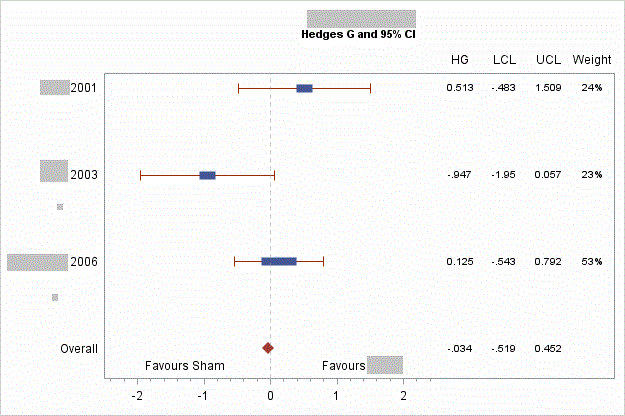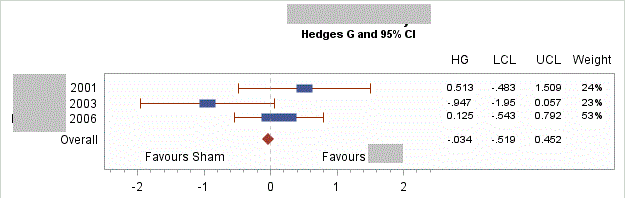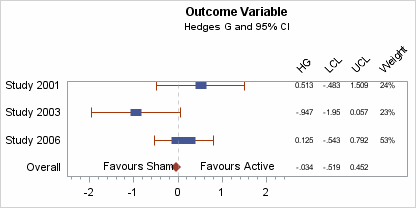## How to decrease the space between studies? (forest plot)

Hi there,

I currently have a few forest plots like this (obscured some details as not sure my employer would want it online):I'd prefer it like this (done in paint):ods graphics / reset width=400px height=200px imagename="Forest_Plot_Vector" imagefmt=gif;

I've tried modifying the above line in the code, but then it renders something like this:Any ideas? Many thanks for any advice

Code used:

``````data forest;
input Study \$1-18 grp OddsRatio LowerCL UpperCL Weight;
format weight percent5. Q1 Q3 4.2 oddsratio lowercl uppercl 5.3;
ObsId=_N_;
OR='HG'; LCL='LCL'; UCL='UCL'; WT='Weight';
if grp=1 then do;
weight=weight*.01;
Q1=OddsRatio-OddsRatio*weight;
Q3=OddsRatio+OddsRatio*weight;
lcl2=lowercl;
ucl2=uppercl;
end;
else study2=study;
datalines;
Study 2001			1	0.513	-0.483	1.509	23.8
Study 2003			1	-0.947	-1.952	0.057	23.4
Study 2006	     	1	0.125	-0.543	0.792	52.9
Overall				2	-0.034	-0.519	0.452	.
;
run;

proc sort data=forest out=forest2;
by descending obsid;
run;

/* Add sequence numbers to each observation */
data forest3;
set forest2 end=last;
retain fmtname 'Study' type 'n';
studyvalue=_n_;
if study2='Overall' then study2value=1;
else study2value = .;

/* Output values and formatted strings to data set */
label=study;
start=studyvalue;
end=studyvalue;
output;
if last then do;
hlo='O';
label='Other';
end;
run;

/* Create the format from the data set */
proc format library=work cntlin=forest3;
run;

/* Apply the format to the study values and remove Overall from Study column. */
/* Compute the width of the box proportional to weight in log scale. */
data forest4;
format studyvalue study2value study.;
drop fmtname type label start end hlo pct;
set forest3 (where=(studyvalue > 0)) nobs=nobs;

/* Compute marker width */
c=0.5; /* Factor to adjust absolute marker width */
x1=oddsratio - c*weight;
x2=oddsratio + c*weight;

/* Compute top and bottom offsets */
if _n_ = nobs then do;
pct=0.75/nobs;
call symputx("pct", pct);
call symputx("pct2", 2*pct);
call symputx("count", nobs);
end;
run;

ods listing close;
ods html image_dpi=100 path="." file='sgplotforest.html';
ods graphics / reset width=400px height=200px imagename="Forest_Plot_Vector" imagefmt=gif;

title "Outcome Variable";
title2 h=8pt 'Hedges G and 95% CI';

proc sgplot data=forest4 noautolegend;
scatter y=study2value x=oddsratio / markerattrs=graphdata2(symbol=diamondfilled size=10);
scatter y=studyvalue x=oddsratio / xerrorupper=ucl2 xerrorlower=lcl2 markerattrs=graphdata1(symbol=squarefilled size=0);
vector x=x2 y=studyvalue / xorigin=x1 yorigin=studyvalue lineattrs=graphdata1(thickness=8) noarrowheads;
scatter y=studyvalue x=or / markerchar=oddsratio x2axis;
scatter y=studyvalue x=lcl / markerchar=lowercl x2axis;
scatter y=studyvalue x=ucl / markerchar=uppercl x2axis;
scatter y=studyvalue x=wt / markerchar=weight x2axis;
refline 0 / axis=x lineattrs=(pattern=shortdash) transparency=0.5;
inset '           Favours Sham'  / position=bottomleft;
inset 'Favours Active'  / position=bottom;
xaxis offsetmin=0 offsetmax=0.35 min=-2.5 max=2.5 minor display=(nolabel) ;
x2axis offsetmin=0.7 display=(noticks nolabel);
yaxis display=(noticks nolabel) offsetmin=0.1 offsetmax=0.05 values=(1 to &count by 1);
run;

ods html close;
ods listing;``````

3 REPLIES 3

## Re: How to decrease the space between studies? (forest plot)

Playing around with height/width should get you there. You need it wider for sure and a bit taller.

## Re: How to decrease the space between studies? (forest plot)

Hi @j4ne,

Sorry for the delayed reply. I've been offline for some time.

In addition to the width and height parameters in the ODS GRAPHICS statement (to adjust the overall size of the graph) you may want to increase the OFFSETMIN and OFFSETMAX values of the YAXIS statement, in order to increase the space between the bottom/top tick mark and the lower/upper end of the y-axis. Example: offsetmin=0.3 offsetmax=0.15.

The font size of the HG, LCL, UCL and Weight values could be increased by adding the MARKERCHARATTRS= option with a suitable font size value such as 10 to the corresponding SCATTER statement. Example (for the Weight values):

scatter y=studyvalue x=wt / markerchar=weight x2axis markercharattrs=(size=10);Jay54
Meteorite | Level 14

## Re: How to decrease the space between studies? (forest plot)

If you have to make the same graph with different data, you can compute the number of categories, and compute a height for the graph into a macro variable and set it on the HEIGHT option.

If the width is too small for the data table on the right, the column headers (that are really X2 axis tick values) will rotate to fit.  To avoid this, ensure the space available for the columns is adequate, by either providing enough graph WIDTH, or enough space for the columns by setting the correct OffsetMin and OffsetMax on the X and X2 axes..

Discussion stats
• 3 replies
• 2506 views
• 2 likes
• 4 in conversation